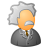# QlikView App Dev

Discussion Board for collaboration related to QlikView App Development.

Announcements
Join us at the Cloud Data and Analytics Tour! REGISTER TODAY
cancel
Showing results for
Search instead for
Did you mean:Creator III

## How to write case statement in Qlikview ?

Hello,

I'm trying to convert the below case statement into QlikView.

Can someone help me with the same?

case

WHEN (value_type = 'P' AND Conv_Value_\$ <= 10) THEN '<10%'

when (value_type = 'P' AND (Conv_Value_\$ > 10 and Conv_Value_\$ <= 14)) THEN  '10-14%'

when (value_type = 'P' AND (Conv_Value_\$ > 14 and Conv_Value_\$ <= 20)) THEN  '14-20%'

when (value_type = 'P' AND (Conv_Value_\$ > 20 and Conv_Value_\$ <= 25)) THEN  '20-25%'

when (value_type = 'P' AND (Conv_Value_\$ > 25 and Conv_Value_\$ <= 30)) THEN  '25-30%'

when (value_type = 'P' AND (Conv_Value_\$ > 30 and Conv_Value_\$ <= 35)) THEN  '30-35%'

when (value_type = 'P' AND (Conv_Value_\$ > 35 and Conv_Value_\$ <= 40)) THEN  '35-40%'

when (value_type = 'P' AND Conv_Value_\$ > 40) THEN '>40%'

Else Null

end as Value_Bucket;

any help greatly appreciated,

thanks

Bhavesh

1 Solution

Accepted SolutionsMVP

if(value_type = 'P' AND Conv_Value_\$ <= 10 ,'<10%',

if(value_type = 'P' AND Conv_Value_\$ > 10 and Conv_Value_\$ <= 14 ,'10-14%',

if(value_type = 'P' AND Conv_Value_\$ > 14 and Conv_Value_\$ <= 20 ,'14-20%',

if(value_type = 'P' AND Conv_Value_\$ > 20 and Conv_Value_\$ <= 25 ,'20-25%',

if(value_type = 'P' AND Conv_Value_\$ > 25 and Conv_Value_\$ <= 30 ,'25-30%',

if(value_type = 'P' AND Conv_Value_\$ > 30 and Conv_Value_\$ <= 35 ,'30-35%',

if(value_type = 'P' AND Conv_Value_\$ > 35 and Conv_Value_\$ <= 40 ,'35-40%',

if(value_type = 'P' AND Conv_Value_\$ > 40) ,'>40%'))))))))

as Value_Bucket;

talk is cheap, supply exceeds demand
4 RepliesMVP

if(value_type = 'P' AND Conv_Value_\$ <= 10 ,'<10%',

if(value_type = 'P' AND Conv_Value_\$ > 10 and Conv_Value_\$ <= 14 ,'10-14%',

if(value_type = 'P' AND Conv_Value_\$ > 14 and Conv_Value_\$ <= 20 ,'14-20%',

if(value_type = 'P' AND Conv_Value_\$ > 20 and Conv_Value_\$ <= 25 ,'20-25%',

if(value_type = 'P' AND Conv_Value_\$ > 25 and Conv_Value_\$ <= 30 ,'25-30%',

if(value_type = 'P' AND Conv_Value_\$ > 30 and Conv_Value_\$ <= 35 ,'30-35%',

if(value_type = 'P' AND Conv_Value_\$ > 35 and Conv_Value_\$ <= 40 ,'35-40%',

if(value_type = 'P' AND Conv_Value_\$ > 40) ,'>40%'))))))))

as Value_Bucket;

talk is cheap, supply exceeds demandChampion III

May be try this?

IF(value_type = 'P' AND Conv_Value_\$ <= 10, '<10%',

IF(value_type = 'P' AND (Conv_Value_\$ > 10 and Conv_Value_\$ <= 14), '10-14%',

IF(value_type = 'P' AND (Conv_Value_\$ > 14 and Conv_Value_\$ <= 20),  '14-20%',

IF(value_type = 'P' AND (Conv_Value_\$ > 20 and Conv_Value_\$ <= 25),  '20-25%',

IF(value_type = 'P' AND (Conv_Value_\$ > 25 and Conv_Value_\$ <= 30), '25-30%',

IF(value_type = 'P' AND (Conv_Value_\$ > 30 and Conv_Value_\$ <= 35), '30-35%',

IF(value_type = 'P' AND (Conv_Value_\$ > 35 and Conv_Value_\$ <= 40), '35-40%',

IF(value_type = 'P' AND Conv_Value_\$ > 40),'>40%', Null()))))))) AS Value_BucketCreator III
Author

thank you, it worked out for meCreator III
Author

thank you, I understood now how to write the case statements in Qlikview